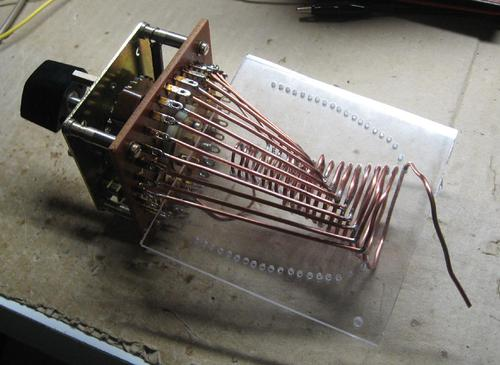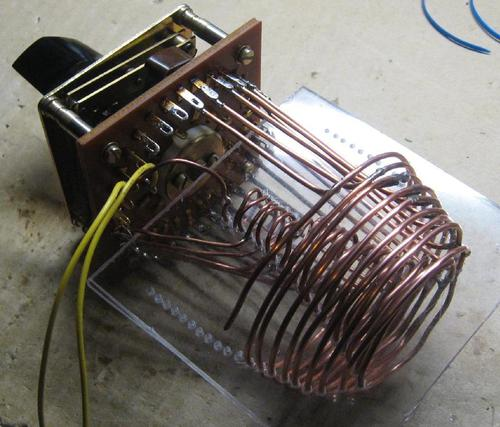# Exponentially switchable coil

Pieter-Tjerk de Boer, PA3FWM pa3fwm@amsat.org

(This is an adapted version of part of an article that I wrote for the Dutch amateur radio magazine Electron, May 2014.)

Many designs for HF antenna tuners incorporate an inductor (coil) that can be varied over a large range, from a fraction of a microhenry to several tens of microhenries. The most common form of such a tunable coil is the roller inductor, but in this article I would like to propose an alternative, in the form of a coil with a set of suitably chosen taps.

## Roller inductor

A roller inductor a long, cylindric coil, combined with a mechanism that allows rolling a tap over the coil, and thus using a larger or smaller part of the coil. If we increase the number of turns of the coil being used, initially the inductance increases quadratically in the number of turns (I wrote a brief explanation of this here). However, as the coil becomes longer, the increase of the inductance slows down, and in the end is only linear in the number of turns. This is because the turns at the start and the end of the coil are so far apart, that they hardly see each other's magnetic field. A roller inductor is usually much longer than its diamater, and thus will mostly exhibit such a linear increase of the inductance in the number of turns.

## Coil with taps

A different way of making a variable inductor, is by using a switch to select one of a set of (fixed) taps on a coil. Clearly, this has the disadvantage that the inductance can only be changed in steps, rather than continuously as with the roller inductor. Thus, we have to make the steps "small enough", but the smaller the steps are, the more positions the switch needs to have. So how small do those steps need to be? Is 1 µH small enough, or do they need to be 0.1 µH ?

It turns out that the required stepsize depends on the inductance itself. If the inductance is small, the steps also must be small, while at larger inductance the steps can also be bigger. This is comparable to resistors: those are manufactured according to e.g. the E12 series, so besides 10 ohm also 12 ohm is for sale, but besides 1000 ohm one can't buy 1002 ohm, because there are only few applications where 2 ohms on 1000 ohms is significant. Every next resistor from the E12 series is 20% larger than its predecessor. Mathematically, this is called an exponential increase. Also in the case of a switchable coil for an antenna tuner, one would wish that with every position of the switch, the inductance increases by a fixed percentage.

If we would make a tap on every turn, then initially, for small inductances, the steps would be way too large, and at the end, for larger inductances, the steps would be unnecessarily small. So in the beginning we should make taps on parts of turns, but that's difficult because of the wiring, because those wires somehow must go to the switch, and that contributes to the inductance as well. And at the end of the coil, we could skip more and more turns.

A different way of adapting the inductance increase per tap, is to make the turns with different diameters. A turn with a small diameter has less inductance than one with a large diameter. So to obtain the desired exponential increase, one should start with small turns, and in the course of the coil make them bigger and bigger, such that every next turn gives a fixed percentage extra inductance. The "only" thing left to do, is calculating the appropriate diameters.

## Inductance calculation

It is not easy to calculate the inductance of a coil of which the turns have varying diameters; there are no ready-made formulas for this. But a computer can calculate this. A suitable program for this is FastHenry. This program takes an input file containing the coordinates of lots of straight pieces of wire (which together form the coil), and then calculates the inductance between two connections to this structure.

In order to experiment with this, I wrote a small auxiliary program which allows me to generate an input file for FastHenry based on a prescribed set of diameters of the turns of a coil. Then I played around with those diameters until, according to FastHenry, the inductance increased in the desired way when selecting different taps. That was quite a puzzle, because changing e.g. the diameter of the 10th turn, does not just affect the increase of the inductance when switching from the 9th to the 10th tap, but also affects the inductance contribution of the 11th and later turns.

## Practical realizationTo build the actual coil, I used a sturdy 32-position rotary switch, bought at an amateur radio fleamarket. The best way to understand the mechanical structure of the coil is by looking at the pictures, which show two intermediate states of the construction and the final object.

The wire of the coil is kept in place by threading it through a piece of plexiglass, in which holes have been drilled at the right places. The first picture shows the coil when half of the turns had been placed; one also clearly sees the wires from each tap to the switch. To keep the whole assembly compact, I designed it such that halfway, the turns "fold back", as can be seen in the second picture. Thus, the last (largest) turn is again near the switch, wide around the first (smallest) turn. The pictures also show that the last few turns have a constant diameter; thus, the size is kept small, but in order to compensate for this, some turns are skipped between the last few taps.

The unused part of the coil is not left unconnected, but short-circuited (by the switch), as is also usual practice in roller coils. There are two reasons for this: if one wouldn't do it, there could be a very high voltage over the unused part of the coil because the whole structure works as a transformer, and in the unused part undesired resonances could occur. Short-circuiting the unused part lowers the inductance slightly, and was therefore taken into account in the FastHenry modelling.

## Measurement resultsFinally, I measured the properties of the coil, and those exceeded my expectations. The plot shows the measured inductance (in red) for each of the switch positions, and the value predicted by FastHenry (in green). The match is very good; the difference of 0.15 µH can be ascribed to the wiring and the switch, which were not included in the FastHenry model. The measured Q (blue line) is above 150 in almost all cases. The coil is now in use in my antenna tuner, and performs quite well in practice.

Unfortunately, it does not make sense to publish a detailed building plan with sizes and a drilling pattern, because it all depends on the available switch. The number of switch positions does not only determine the number of taps, but also the mechanical details. If there's a suitable and widely available switch, I might be convinced to repeat the calculations for that switch so the design can be copied...

Text and pictures on this page are copyright 2014, P.T. de Boer, pa3fwm@amsat.org .
Republication is only allowed with my explicit permission.